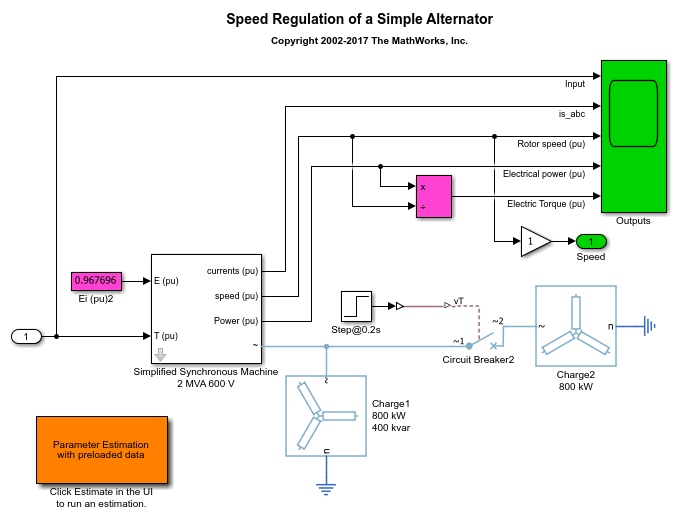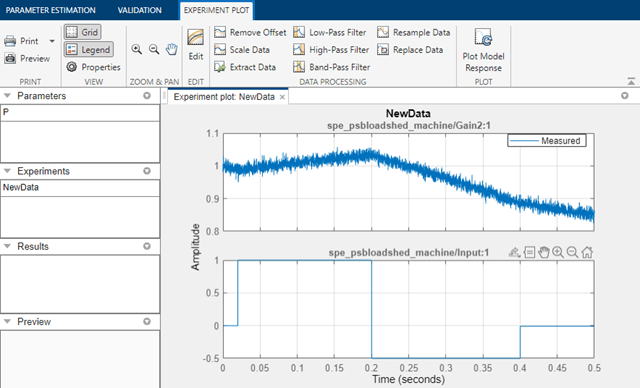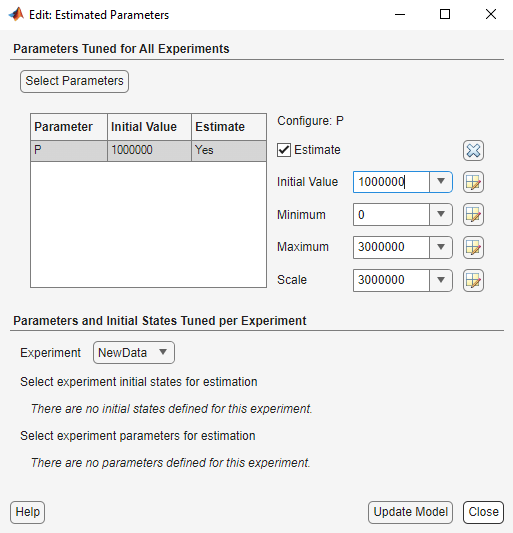# Simplified Alternator Parameter Estimation

This example shows how to use parameter bounds to improve estimation performance. This is illustrated by estimating the power rating, P, of a synchronous machine.

This example requires Simscape™ and Simscape Electrical™.

### Simulink Model of the Simplified Alternator

The Simulink® model for the alternator system, `spe_psbloadshed_machine`, is shown below.### Model Description

A three-phase, four-wire alternator rated 2000 kVA, 1600 kW, 0.8 power factor, 600 V, 1800 rpm is connected to a 1600 kW, 400 kvar inductive load. The stator neutral point is grounded. The internal impedance of the generator (Zg = 0.0036 + j*0.16 pu) represents the armature winding resistance Ra and direct axis transient reactance X'd. The total inertia constant of the generator and prime mover is H = 0.6 s, corresponding to J = 67.5 kg.m^2.

A three-phase breaker is used to switch out a 800 kW resistive load. The breaker is initially closed and it is opened at t = 0.2 s, resulting in a 50% load shedding.

The machine is excited with a constant voltage. The mechanical torque is modeled as a two-step signal. The first step has a magnitude of 1.0 and a duration of 0.18s. The second step has a magnitude of -0.5 for a duration of 0.2s.

### Estimation Data

Double-click the orange block on the left side of the model to open the Parameter Estimator already loaded with experimental data, `NewData`. The input and output data from this experiment are shown in the plot. There is only one data set used for this example.### Define Variables

Click Select Parameters in the Parameter Estimation tab to specify parameters for estimation. Here, we have already loaded the parameters for this model. There is only one parameter in this model that we are interested in estimating, the nominal power, `P`, of the Simplified Synchronous Machine.

We know from the specs that this value should be around 2000kW but here we assume that we only have an initial guess of 3000kW. We also set the minimum value of the nominal power to be 3000kW, and the maximum value to be 4000kW.With the parameters for estimation specified, we select experiments to use for estimation. Click Select Experiments in the Parameter Estimation tab and select `NewData` for estimation.

Click Estimate to start the estimation. The estimation will keep iterating the parameter value and the experiment plot will continue to update, until the estimation converges and terminates.

The plot below shows the experimental data overlaid with the simulated data. The simulated data comes from the model with the estimated parameters. As expected the estimation terminated quickly and the results were not so good because of our initial guess and bounds of the parameter `P`.### Running the Estimation with a Different Initial Guess

Since we are not satisfied with our results, we will choose a different initial guess. This is quite simple to do with the Simulink® Design Optimization™ Parameter Estimator. Click Select Parameters in the Parameter Estimation tab and change the value to 1000 kV and the Minimum and Maximum bounds to 0 kV and 3000 kV respectively.Click Estimate to run another estimation. Once the estimation is complete we can verify that our results are more accurate by looking once again at the measured vs. simulated response.### Conclusion

Placing inappropriate bounds on your parameters can lead to inaccurate results and most often the estimation will not converge successfully. However, with the parameter estimation GUI, it is simple to change these bounds to run another estimation without creating a new estimation project.

Close the model.